Next: About this document ... Up: Ensemble Learning for Independent Previous: References

# Derivations

An approximation for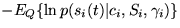is derived here. The derivation makes use of the Jensen's inequality and second order Taylor's series expansion.

Let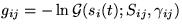. The expectation to be approximated is then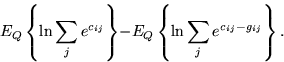Let us first concider the latter term. The logarithm of the sum is a strictly convex function of gij. By Jensen's inequality, moving the expectation inside a convex function cannot result in increment. Replacing the latter expectation by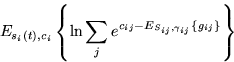can therefore only result in increment in the approximation. This is safe because we are trying to minimise the Kullback-Leibler information and approximating it above is thus conservative.

The expectation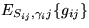is similar to equation 1 and equals to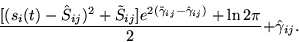Let us denote this by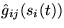. At this point, the approximation equals to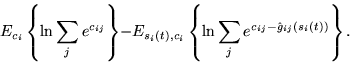The terms inside the expectations are functions of si(t) and ci.

Next, let us concider a second order Taylor's series expansion about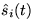and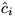. Notice that the first order terms and all second order crossterms disappear in the expectations and only the constant and the pure second order terms remain. This is because the variables are independent in the ensemble.

The constant term will be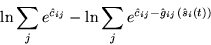(2)
and the remaining second order terms of cij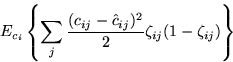and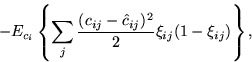where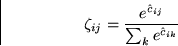and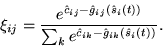Taking the expectations yields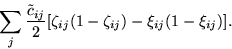(3)
The second order term of si(t) will be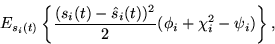where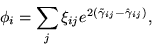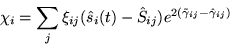and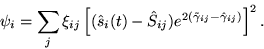Taking the expectation yields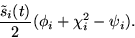(4)
At this point, the approximation of the original expectation is thus the sum of terms in equations 2-4.

Some care has to be taken with the approximation resulting from the Taylor's series expansion because it utilises only local information about the shape of the posterior pdf. For instance, if the meanhappens to be in a valley between two Gaussians, the term in equation 4 will be negative. It then looks like the Kullback-Leibler information can be decreased by increasing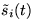. This only holds for small, however. At some point after the distribution of si(t) has become broader than the separation between the two Gaussians, the Kullback-Leibler information starts to increase asincreases.

In order to avoid the problem, only positive terms of equations 3 and 4 will be included. The final approximation is thus equation 2 plus the positive terms of equations 3 and 4.Next: About this document ... Up: Ensemble Learning for Independent Previous: References
Harri Lappalainen
7/10/1998